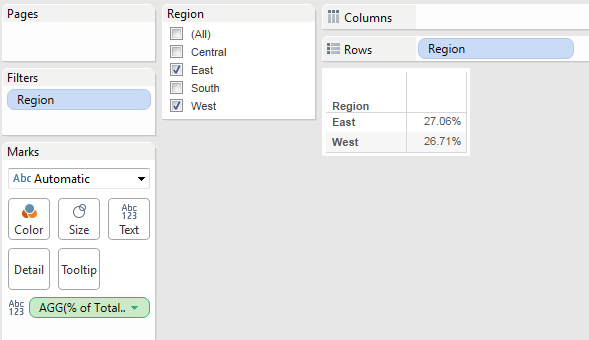# Tableau - Real Time Scenario 7( How to make % of Total Sales consistent after applying filter)

Problem: How to make % of Total Sales consistent after applying filter.In above image showing region wise percentage of total Sales. Before applying filter you can see percentage of total sales for each region. But when apply Region filter only for Central and West. The percentage of total sales changed. This is not desired functionality. I just want to filter rows not values.

You can see my required output in image. How to do this?

Solution:

we can give solution in two ways.

1. using lookup( ): This is not 100% perfect solution.

2. Using Fixed( ): I am providing solution here using Fixed( )

Step 1: Create a calculated field as “Fixed Sales” using below formula.

{FIXED sum([Sales])}

Step 2: Create a calculated field as “% of Total Sales” using below formula

sum([Sales])/sum([Fixed Sales])

Step 3: Drag Region to row shelf and % of Total Sales to Text shelf. Drag Region to filter shelf and show it as quick filter. See belowStep 4: Right click on Agg(% of Total Sales), go to format options, change number format to percentage.

Step 5: Now you will see sales in percentage. Change the filter and see that percentage is not changing.See Report Below: